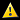# Computing: Free Pascal Programming

## My Free Pascal Console Programs.

Program list:

Domain Program Language Description Exec Source
Biology Hardy-Weinberg Law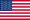Population genetics: Calculating the Hardy-Weinberg equilibrium frequencies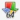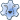Chemistry Chemical equationsSimple command line program to balance chemical equationsComputing Downloads countSimple command line program to determine the count of the monthly downloads from a websideLockscreen picturesVery simple command line program to copy the Windows 10 lockscreen pictures to a user directoryText file mergeSimple text file merge command line programText file sortSimple text file sort command line programGames Simple BlackjackSimple Blackjack command line card gameSnake gameUse the cursor keys to move the snake, that grows by eating meats and dies when hitting rocks (console)Mathematics Arithmetic baublesArithmetic problems for primary school pupils (console version)Burning Ship fractalBurning Ship fractal program. Very simple (text based X-/Y-scaling and zooming), but nice picturesComplex equationsFirst degree complex equations in 1 variableComplex equations IISecond degree complex equations in 1 variableComplex rootsDetermination of all nth roots of a complex numberCubic equationsResolution of cubic equations in one variable (ax3 + bx2 + cx + d = 0)de Jong attractorsMathematical images: Peter de Jong attractorsJulia setsQuadratic Julia sets program. Very simple (text based X-/Y-scaling and zooming), but nice picturesLinear equationsResolution of linear equation systems in one, two or three variablesLines in the plane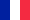Geometry in the plane: Line passing through 2 pointsLines in the plane IIGeometry in the plane: Parallel lines; lines intersection pointMandelbrot setMandelbrot set program. Very simple (elementary scaling and zooming), but nice picturesQuadratic equationsResolution of quadratic equations in one variable (ax2 + bx + c = 0)Various 3-month calendarMulti-lingual calendar with 3 months display. Use cursor and PF keys to change month, year or languageMorse alphabetCharacter or complete text translation (from or to morse code). Morse alphabet knowledge test